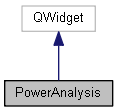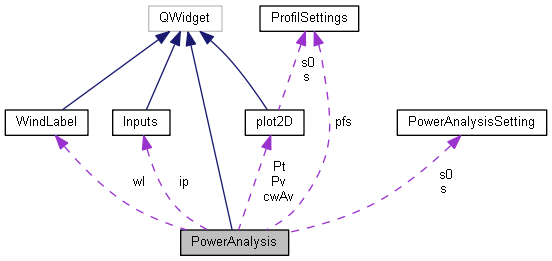$$\def\bold#1{\bf #1} \newcommand{\d}{\mathrm{d}}$$ BTP: Manual and Source Code Documentation

#### Power Uphill

bike mass [kg]
body mass [kg]
altitude gain [m]
climb length [km]
time [s]
speed [km/h]
power [W]
power/mass [W/kg]
climbrate [m/min]

#### average power on climb stageBTP  3.0 Routing/ClimbAnalysis/PowerCalculation
PowerAnalysis Class Reference

power calculation for height-distance datasets More...

#include <poweranalysis.h>

Inheritance diagram for PowerAnalysis:[legend]
Collaboration diagram for PowerAnalysis:[legend]

void recalced ()

## Public Member Functions

PowerAnalysis (PowerAnalysisSetting *s)

void init ()

void set_default (PowerAnalysisSetting *s)
apply default setting to the PowerAnalysisSetting s

void calc_track (Track *t)
calc power from P(v) distribution

void calc_track (Track *t, double *timed, double *time, int count)
calc power from known velocities

double ** get_vdata ()
returns data array pointer

double ** get_Pdata ()
returns data array pointer

int get_datacount ()
returns data array length

double get_t (double d)
interpolate time in data

double get_v (double d)
interpolate time in data

double get_P ()
return average power

double get_v ()
return average velocity

double get_P (double d)

double get_v (double dmin, double dmax)
return average velocity on track section defined by distance points

double get_P (double dmin, double dmax)
return average velocity on track section defined by distance points

## Private Slots

void set_default_refresh ()
reset PowerAnalysisSetting s

void draw_Pv ()
draw P(v) distriubtion

void draw_cwAv ()
draw cwA(v) distriubtion

void update_Pvl (double v)
update info label

void update_cwAl (double v)
update info label

void show_slopespeed_table ()

double bft_to_mpros ()
wind speed in m/s from beaufort

void recalc ()

void toggle_fightwind ()
switch wind handling in fightwind of PowerAnalysisSetting More...

void toggle_timestamp ()
makes poweranalysis ignoring or subordinate time stamp More...

## Private Member Functions

double vmax (double slope, double direction)
physics: calculate the resulting velocity from P(v) at specific slope

double veff (double v, double direction)
physics: total air speed from movement speed v and wind

double rho (double h)
physics: air pressure dependend from height (barometric formula)

double T (double h)
physics: temperature from height with 0.7 K/100m temperature decrease

double P (double v)
velocity depend output power, parametrised with fermi function

double P (double v, double slope, double direction, double rho0)
necessary power to keep velocity, depending on enviroment

double cwA (double v)
velocity depend cwA value, parametrised with fermi function

void track_to_data (Track *t)
extracs calculation data

void calc_power ()
execute the calculation

## Private Attributes

double vwind
wind velocity

WindLabelwl
wind visualization

QCheckBox fwcb
fight wind checkbox

Inputsip 
user interface

PowerAnalysisSettings
assigned settings to be used

PowerAnalysisSetting s0
intrinsic settings

plot2D Pt
calculated power-time distriubtion

plot2D Pv
user defined power output

plot2D cwAv
user defined cwA value

QLabel Pvl

QLabel cwAvl
plot2d info area

QPushButton bdefault

QPushButton brecalc

QPushButton btoggletimestamp

double * plotdata 
container to collect plotting data

ProfilSettings pfs

QPlainTextEdit textbox

double * d 

double ds

double Ebrake

double Eout

double * Pdata 

double * vdata 

int count
data array length

bool timeexisting
is time data existing?

## Detailed Description

power calculation for height-distance datasets

This class provides a trivial integration routine to calculate the power output of a self powered object (e.g. a cyclist) on a track. The cyclist is defined by mass, cwA, coefficient of friction, maximum acceleration in bends and for breaking. A velocity dependend power output P(v) is holds for the whole calculation. The enviroment is defined by its pressure at sea level its temperature und wind strength and direction. The track is an dataset holding the height and distance data as well as coordinates to calculate bend radius to estimate maximum velocity to pass the bends and possibly force a breaking. All the parameters are specified in PowerAnalysisSetting.

The calculation itself is trivial: the current cyclista Power output (depending on the current velocity) minus the the current resitences (aerodynamic drag, road resistance, climb power) leads to the total acceleration which can of course be negative. If the requires breaking then Power output is set to zero and the required breaking power is applied. Loading an time data as well the calculation is no more based on the Power output P(v) but on the realized velocity.

Definition at line 76 of file poweranalysis.h.

## Member Function Documentation

 double PowerAnalysis::get_P ( double d )

return power at distance point d

Definition at line 566 of file poweranalysis.cpp.

 void PowerAnalysis::recalc ( )
privateslot

perform the whole calculation

Definition at line 626 of file poweranalysis.cpp.

 void PowerAnalysis::toggle_fightwind ( )
privateslot

switch wind handling in fightwind of PowerAnalysisSetting

the cyclist is allowed in two ways to react on hitting wind, this is: a) fight the wind, i.g. serve a higher Power than usually apllied at this speed. This higher Power is calculated by vector additon of wind and moving velocity thus imitating that speed was even lower b) adapt power output P(v) to the real moving velocity, i.g. Power output is only raised due to the reduce velocity (which should be related to a higher Power output)

Both principles apply at tailwind, i.g. in case a) relaxing even more than in case b). To put it in Nutshells: Fighting the wind will lead to less velocity deviations from the average.

Definition at line 630 of file poweranalysis.cpp.

 void PowerAnalysis::toggle_timestamp ( )
privateslot

makes poweranalysis ignoring or subordinate time stamp

if a time stamp exists from a gpx or was previously loaded, than the rider specific, velocity dependend power output is ignored and power is calculated to satisfy the give velocity time with respect to the PowerAnalysisSetting. If this time stamp should be ignored, then the time is calculated from the rider specific power output.

Definition at line 636 of file poweranalysis.cpp.

## Member Data Documentation

 double* PowerAnalysis::d
private

data array 0: distance [meter] 1: height [meter] 2: azimut 3: bend 4: vmax due to bend 5: calculated v 6: seconds 7: Power 8: Energy

Definition at line 182 of file poweranalysis.h.

The documentation for this class was generated from the following files: